Question

5. Figure 5 shows an electrical network, i) obtain the differential equations of the network and nofind the tran...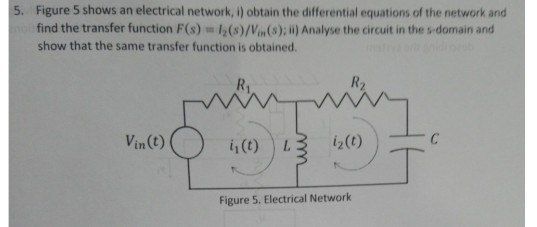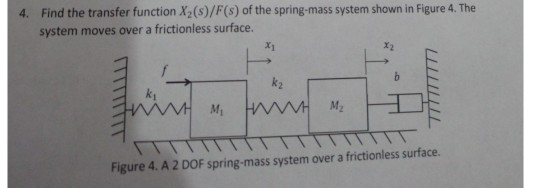5. Figure 5 shows an electrical network, i) obtain the differential equations of the network and nofind the transfer function F(s) show that the same transfer function is obtained 12(s)/Vin(s); ii) Analyse the circuit in the s-domain and R. C i2(t) Vin(t) L Figure 5. Electrical Network
4. Find the transfer function X2(s)/F(s) of the spring-mass system shown in Figure 4. The system moves over a frictionless surface. h M2 M1 Figure 4. A 2 DOF spring-mass system over a frictionless surface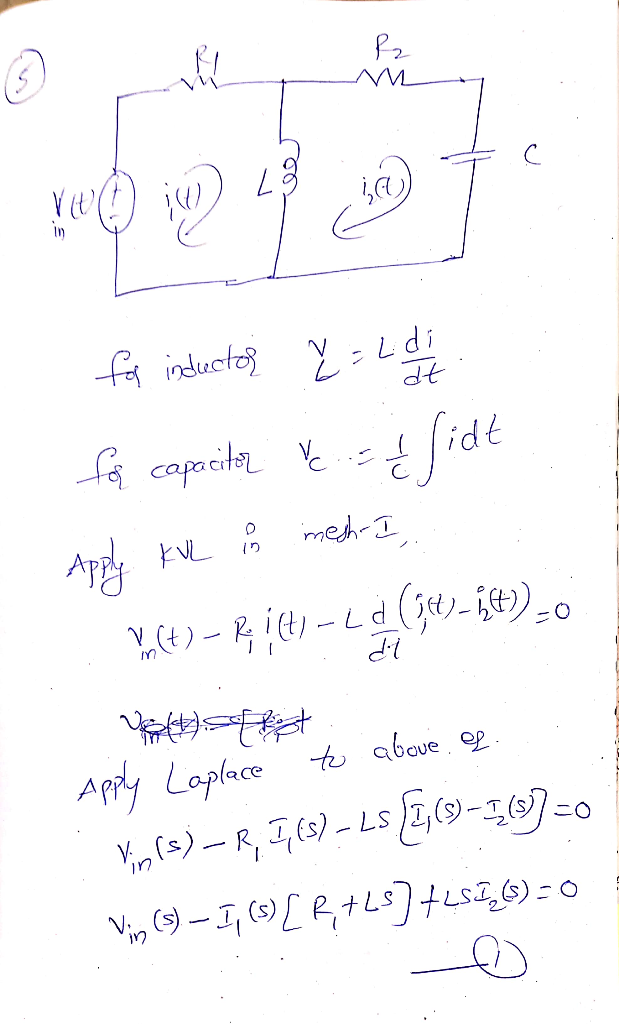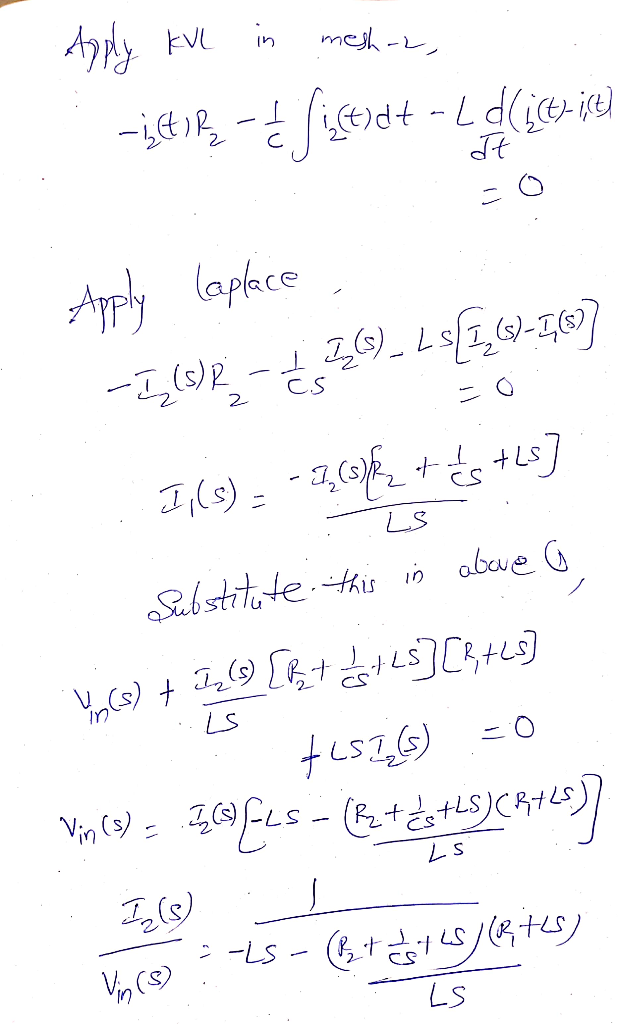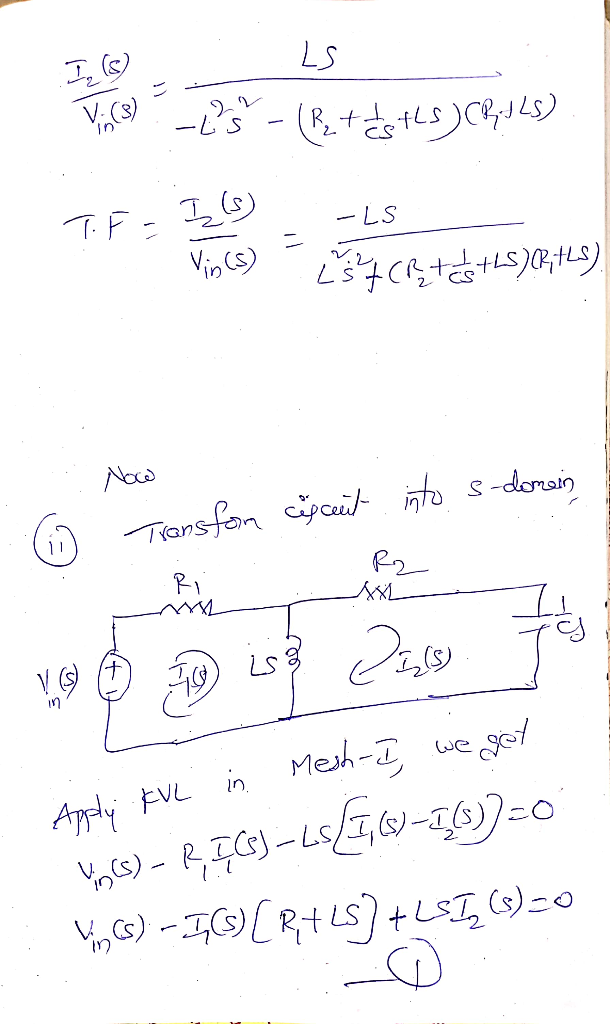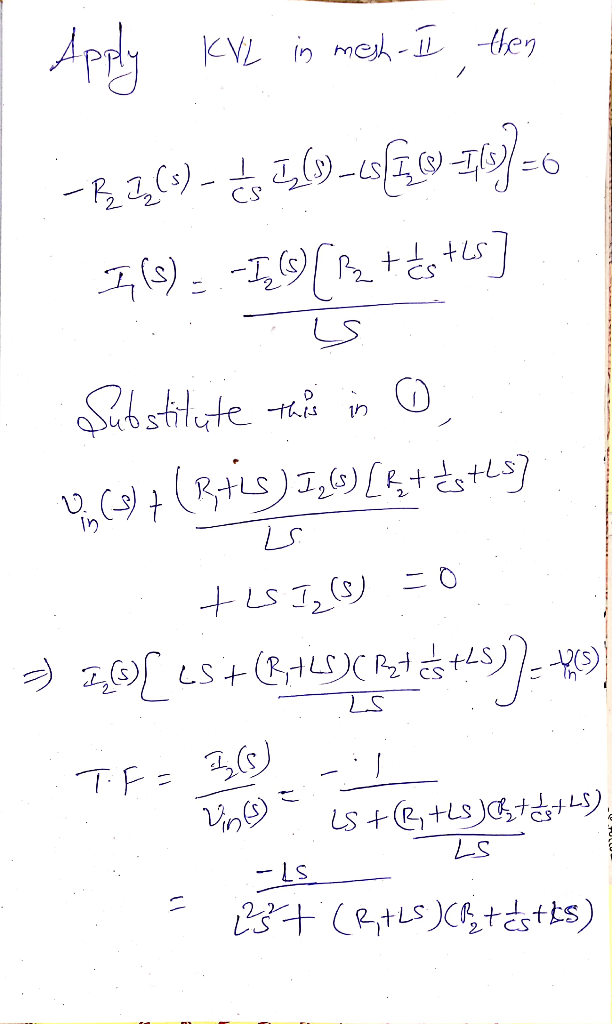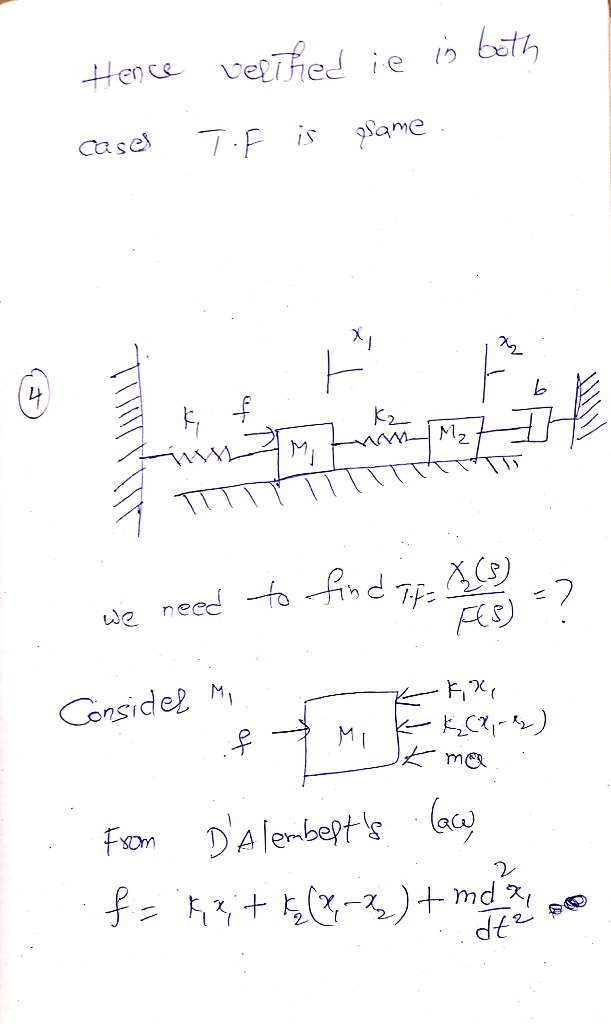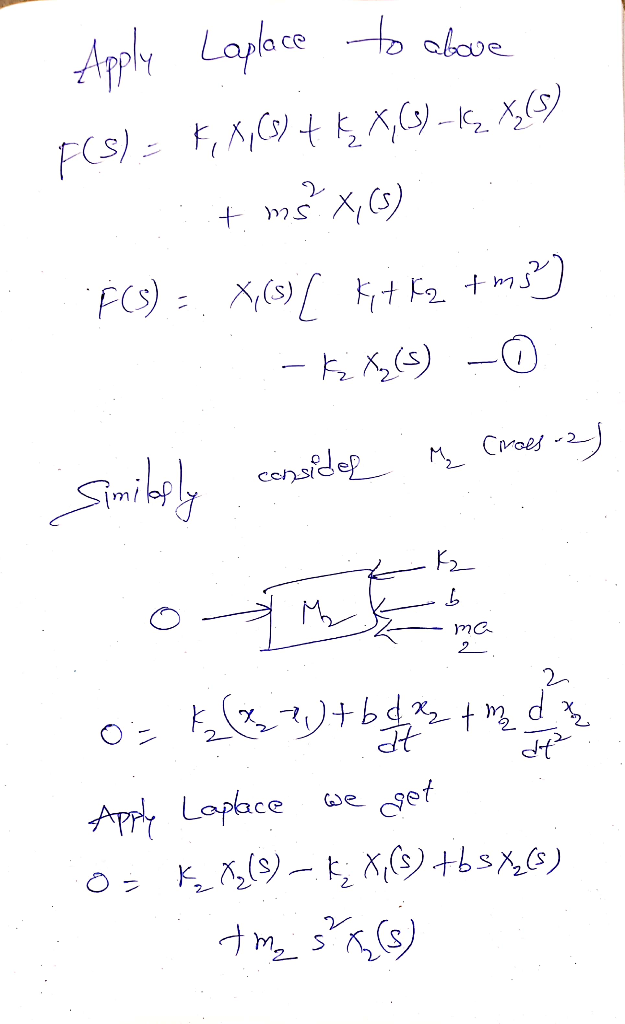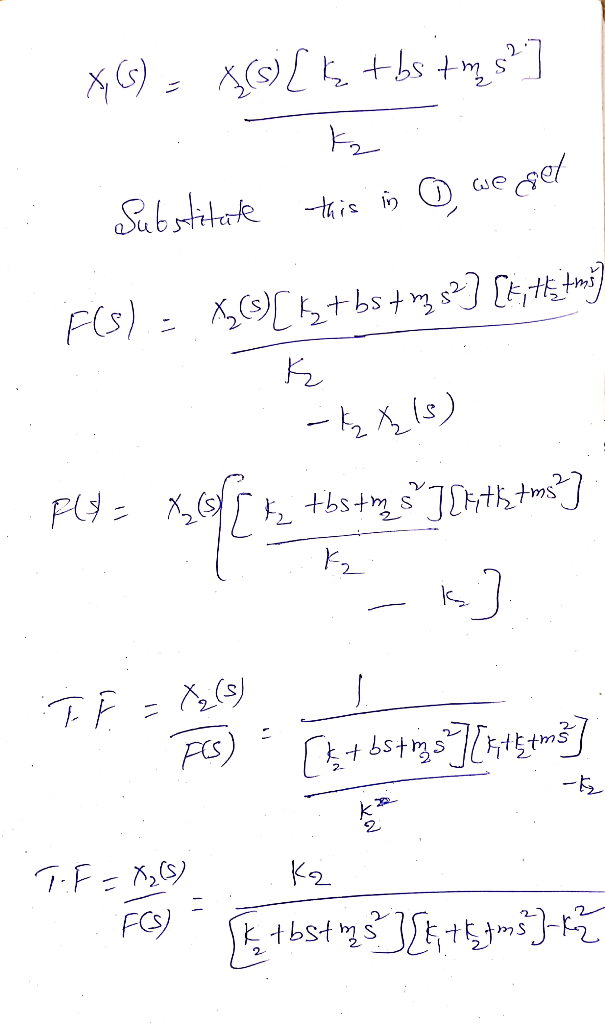Earn Coins

Coins can be redeemed for fabulous gifts.

Similar Homework Help Questions
• 3. A motor with torque T and inertia Jm drives a load of inertia Joad via a shaft which may be assumed to have infin...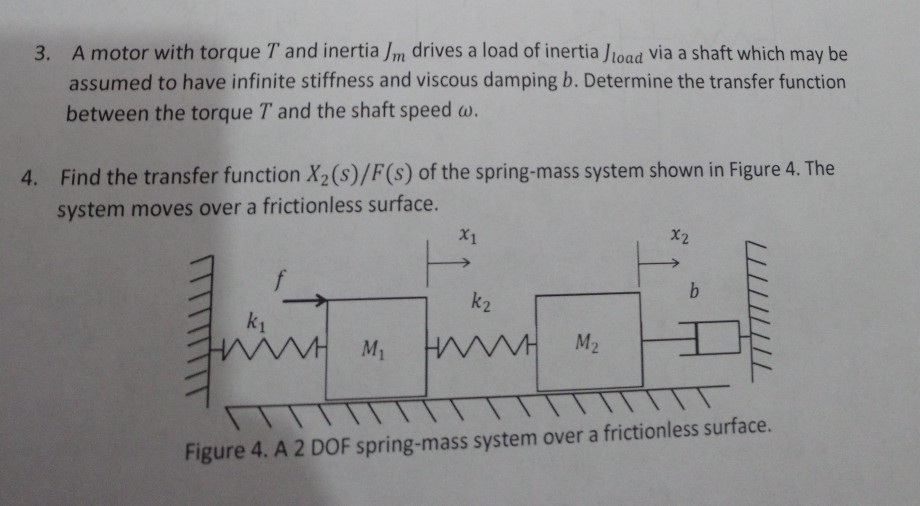3. A motor with torque T and inertia Jm drives a load of inertia Joad via a shaft which may be assumed to have infinite stiffness and viscous damping b. Determine the transfer function between the torque T and the shaft speed w. 4. Find the transfer function X2(s)/F (s) of the spring-mass system shown in Figure 4. The system moves over a frictionless surface X2 X1 b k2 k1 M2 M1 Figure 4. A 2 DOF spring-mass system over...

• The system of differential equations for the currents i1 (t) and i2(t) in the electrical network...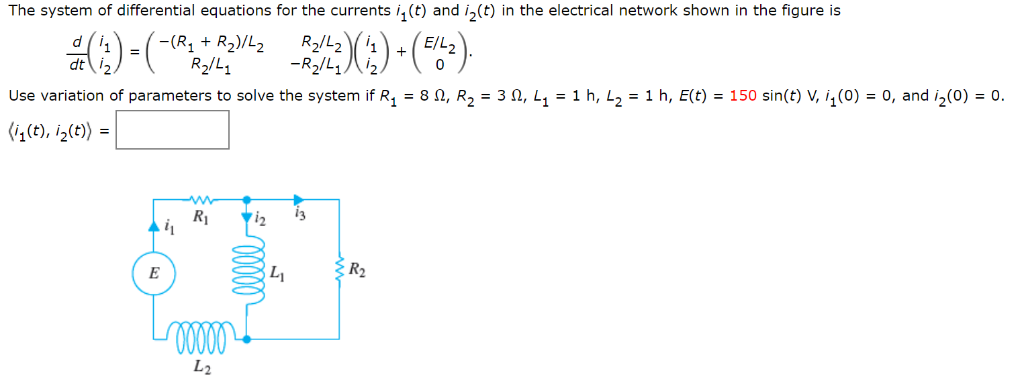The system of differential equations for the currents i1 (t) and i2(t) in the electrical network shown in the figure is dt(々 =( R2-212/ R2/L1 Use variation of parameters to solve the syster if R1 = 8 Ω, R2-3 Ω, L1 = 1 h, L2-1 h, E(t) = 150 sin(t) V i1(0) = 0, and i2(0) = 0. (i1 (t),ら(t) = R2 し2

• a) [15 marks] Write the differential equations that describe the behavior of the electrical system shown...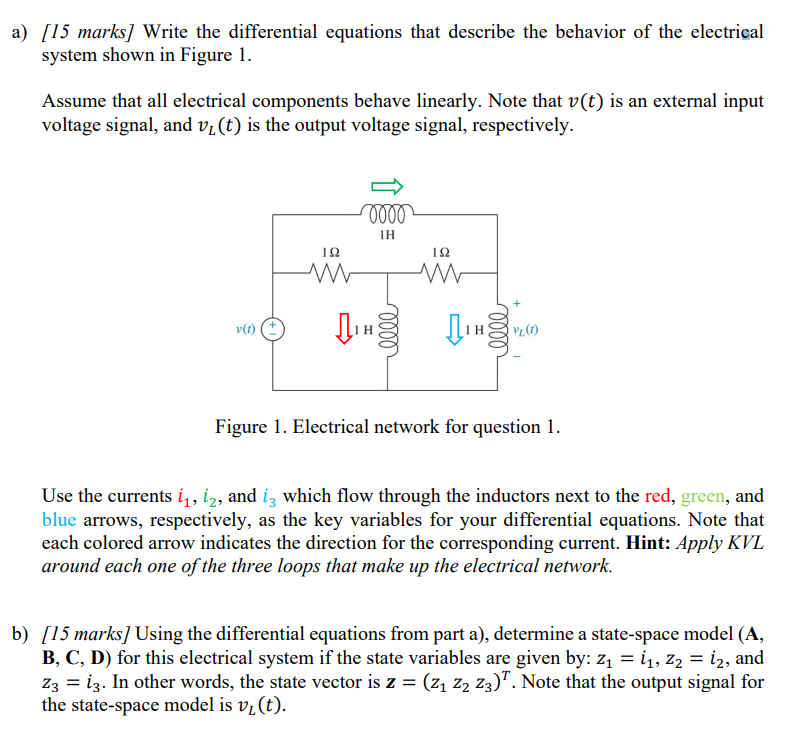a) [15 marks] Write the differential equations that describe the behavior of the electrical system shown in Figure 1. Assume that all electrical components behave linearly. Note that v(t) is an external input voltage signal, and vi(t) is the output voltage signal, respectively. 0000 1H 1Ω 1Ω M v(t) Figure 1. Electrical network for question 1. Use the currents ij, iz, and iz which flow through the inductors next to the red, green, and blue arrows, respectively, as the key...

• Q2. Derive the governing differential equations for the systems shown in the following figures. fv=4 N-s/m K=5 N/mfv =...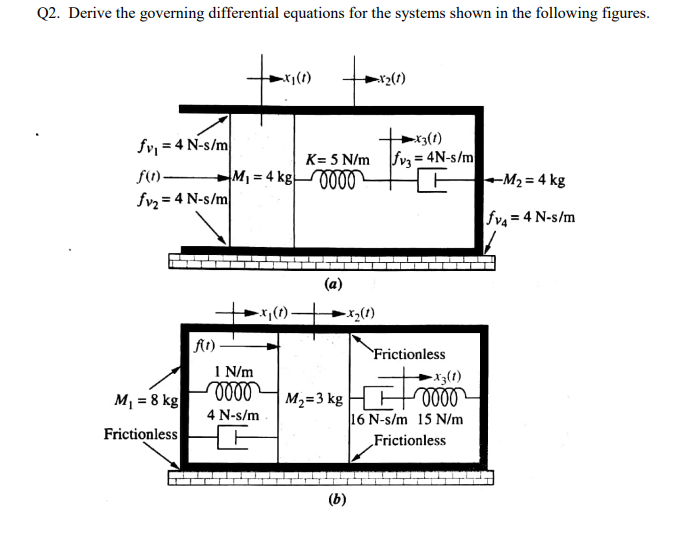Q2. Derive the governing differential equations for the systems shown in the following figures. fv=4 N-s/m K=5 N/mfv = 4N-s/m 000 M1 4 kg f)_ M2 4 kg fv2 4 N-s/m fv= 4 N-s/m (a) fit) Frictionless N/m -xj(t) O000 16 N-s/m 15 N/m 0000 4 N-s/m M1 8 kg M2-3 kg Frictionless Frictionless (b) Q2. Derive the governing differential equations for the systems shown in the following figures. fv=4 N-s/m K=5 N/mfv = 4N-s/m 000 M1 4 kg f)_...

• winkngs spring i(t) v(t) st VEE Figure 1: (a)Solenoid with retu spring. (b) Equivalent lumped electrical cireuit (...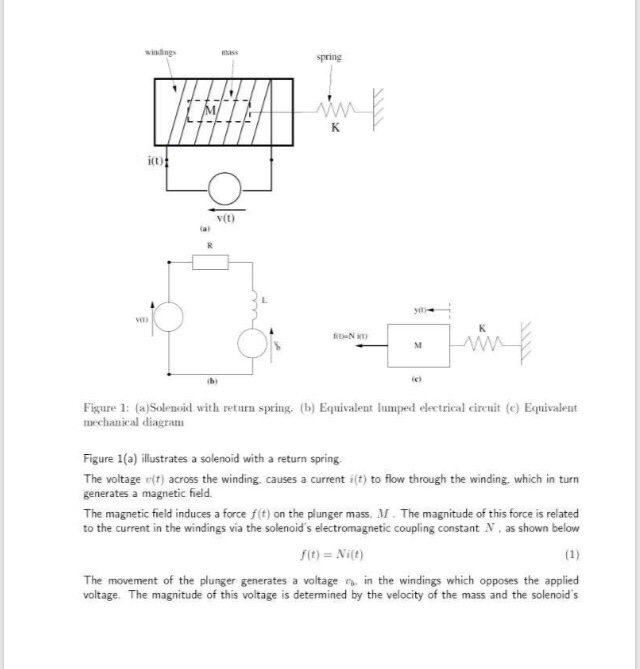winkngs spring i(t) v(t) st VEE Figure 1: (a)Solenoid with retu spring. (b) Equivalent lumped electrical cireuit (e) Equivalent mechanical diagram Figure 1(a) illustrates a solenoid with a return spring The voltage e(t) across the winding, causes a current it) to flow through the winding. which in turn generates a magnetic field The magnetic field induces a force f(t) on the plunger mass, . The magnitude of this force is related to the current in the windings via the solenoid's...

• Problem 4 (50 pts): Consider the electrical circuit represented in figure, with R = 102, C...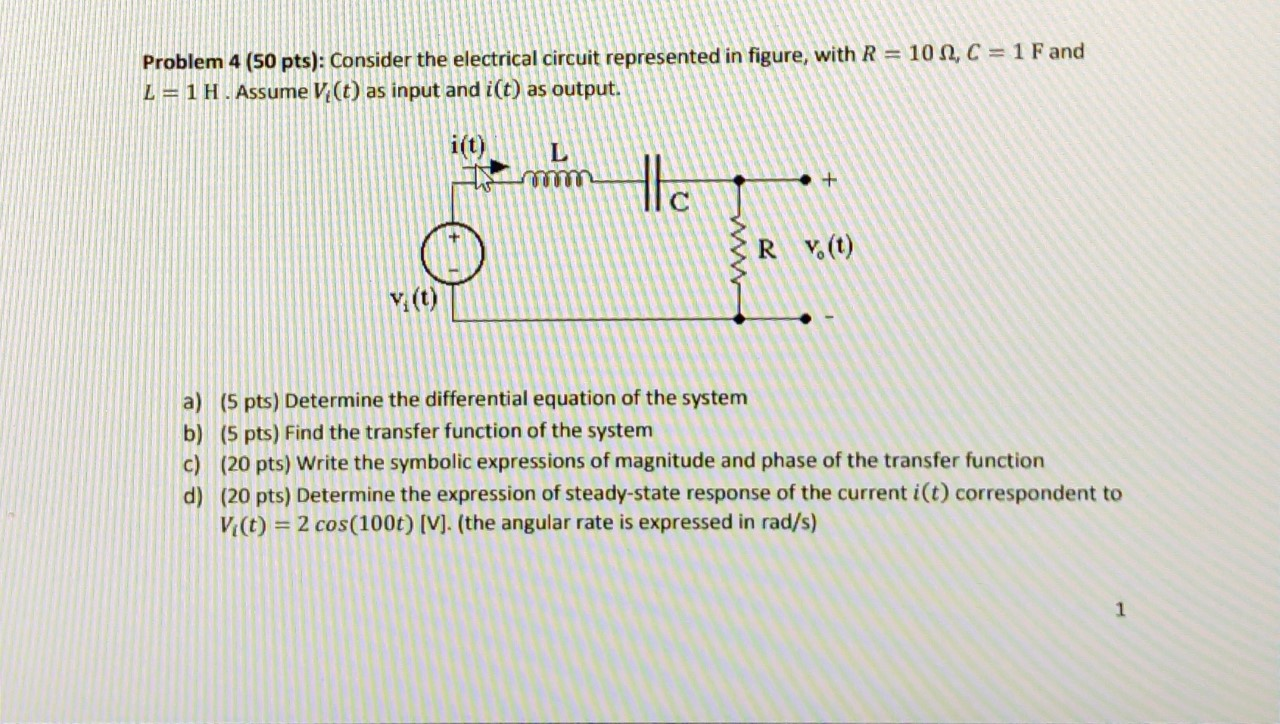Problem 4 (50 pts): Consider the electrical circuit represented in figure, with R = 102, C = 1 F and L=1 H. Assume V (t) as input and i(t) as output E ER V.(t) a) (5 pts) Determine the differential equation of the system b) (5 pts) Find the transfer function of the system c) (20 pts) Write the symbolic expressions of magnitude and phase of the transfer function d) (20 pts) Determine the expression of steady-state response of the...

• Example 5a: Write, but do not solve the equations of motion for the mechanical network of Figure ...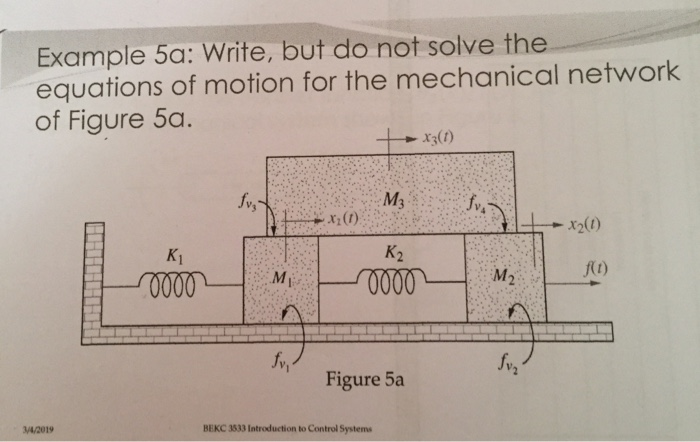translational rotational Example 5a: Write, but do not solve the equations of motion for the mechanical network of Figure 5a. 石 x20) K1 f(t) fv, Figure 5a 4/2019 ВЕКС 3533 Introduction to Control Systems Example 7: Write but do not solve, the Laplace transform of the equations of motion for the system shown in Figure 7. 6,(t) Di D2 D3 Figure 7 Example 8: Find the transfer function, θ2(s)/T(s), for the rotational mechanical system shown in Figure 8. 1 N-m/rad...

• You have two equal masses m1 and m2 and a spring with a spring constant k....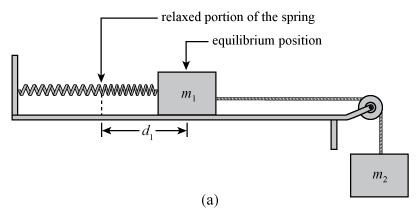You have two equal masses m1 and m2 and a spring with a spring constant k. The mass m1 is connected to the spring and placed on a frictionless horizontal surface at the relaxed position of the spring. You then hang mass m2,  connected to mass m1 by a massless cord, over a pulley at the edge of the horizontal surface. When the entire system comes to rest in the equilibrium position, the spring is stretched an amount d1 as shown...

• Homework problem! Need help fast Example 2: Figure below shows the principle of a vibration damper....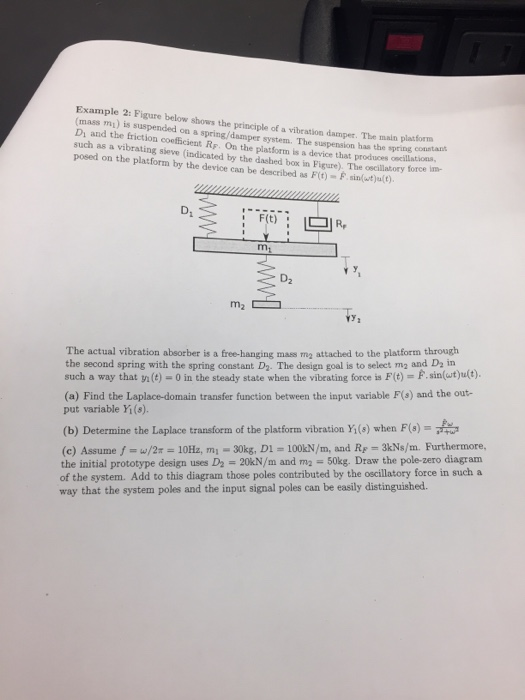Homework problem! Need help fast Example 2: Figure below shows the principle of a vibration damper. The main platform (mass mi) is suspended on a spring/damper system. The suspension has the spring conn Di and the friction coefficient RF. On the platform is a device that produces oscillatioss such as a vibrating sieve (indicated by the dashed box in Figure). The oscillabory force im- posed on the platform by the device can be described as Fit)-Fn(t) D. m. D2 The...

• chapter 13 problem..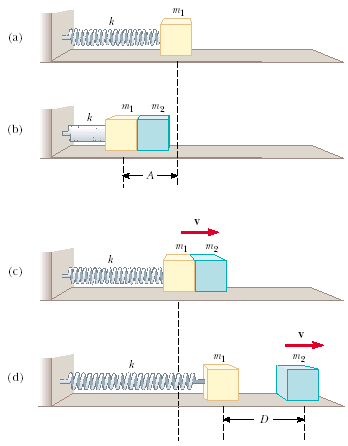An object of mass m1 = 9.9 kg, is in equilibrium while connected to alight spring of constant k = 100 N/m that is fastened to awall asin Figure P13.67a. A second object of massm2 = 6.7 kg, isslowly pushed up against mass m1, compressingthe spring by theamount A = 0.18 m, as shown in Figure P13.67b. The system isthen released, causing both masses to start moving to the right onthe frictionless surface.Figure P13.67(a) When m1 reaches theequilibrium point, m2...